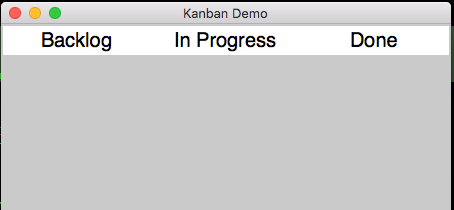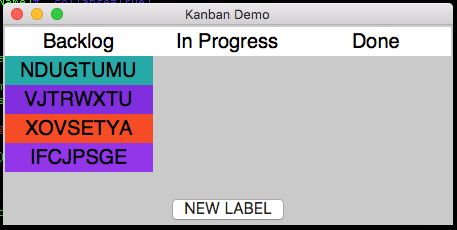# Kanban Demo

A common interface is a to-do list, made up of multiple columns, with the ability to add items to the first column, then move items across the columns. We can create something similar in appJar by laying out labels in columns.

## The Layout

We'll start by creating the GUI with three labels across the top:``````from appJar import gui

ttl = ('Backlog', 'In Progress', 'Done')

with gui("Kanban Demo", "450x200", bg='grey', sticky='new', font=20) as app:
for pos in range(len(ttl)):
app.label(ttl[pos], pos=(0, pos), bg='white', width=12, stretch='column')
``````

Then, we'll add three empty frames and a button, underneath:``````from appJar import gui
from random import choice

ttl = ('Backlog', 'In Progress', 'Done')

def genNewLbl():
with app.frame(ttl):
text = ''.join(choice('ABCDEFGHIJKLMNOPQRSTUVWXYZ') for c in range(8))
app.label(text, bg=app.getRandomColour())

with gui("Kanban Demo", "450x200", bg='grey', sticky='new', font=20) as app:
for pos in range(len(ttl)):
app.label(ttl[pos], pos=(0, pos), bg='white', width=12, stretch='column')
app.stretch = 'both'
with app.frame(ttl[pos], row=1, column=pos, sticky='new'): pass

app.button("NEW LABEL", genNewLbl, colspan=3, sticky='s', stretch='column', font=16)
``````

## Moving the Labels

Next we need to make the labels interactive, so that when they are clicked, they are moved to the next column.

We'll define a function to move a label to the named frame:

``````def move(lName, fName):
# move the label to the new frame
with app.frame(fName): app.moveLabel(lName)
``````

Now, we just need some way for each label to know it's destination frame.

We'll create three wrapper functions to do this:

``````def move1(lName): move(lName, ttl)
def move2(lName): move(lName, ttl)
def trash(lName): app.removeLabel(lName)
``````

Then, we update the function that generates labels to give each one a `submit` function, that moves it to the next column:

``````def genNewLbl():
with app.frame(ttl):
text = ''.join(choice('ABCDEFGHIJKLMNOPQRSTUVWXYZ') for c in range(8))
app.label(text, bg=app.getRandomColour(), submit=move1) # add submit function
``````

And update the move function so that it changes a label's `submit` function when it is moved:

``````def move(lName, fName):
# move the label to the new frame
with app.frame(fName): app.moveLabel(lName)
# change the label's submit function
if fName == ttl: app.label(lName, submit=move2)
else: app.label(lName, submit=trash)
``````

And that's it, we have a way of moving tasks from one column to the next...